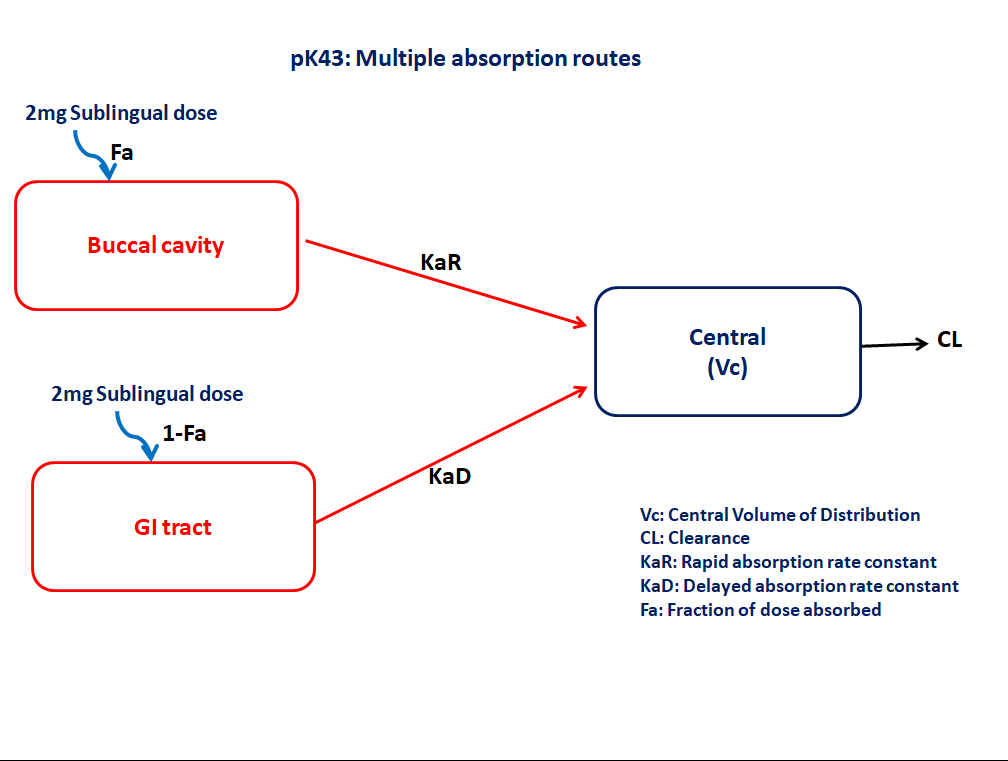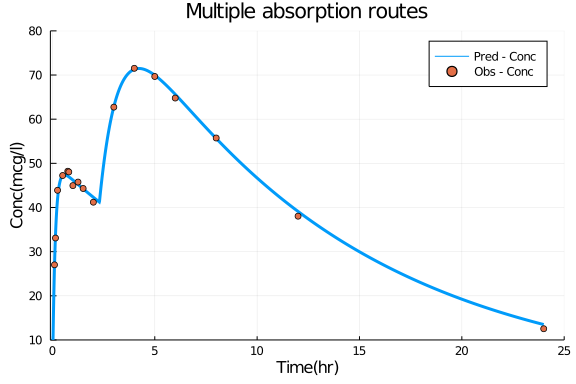# Exercise PK43 - Multiple absorption routes

### Background

• Structural model - 1 compartment linear elimination with first order absorption

• Route of administration - Sublingual

• Dosage Regimen - 2mg Sublingual dose

• Number of Subjects - 1### Learning Outcome

In this model, Multiple absorption routes helps in understanding absorption of sublingually administered dose partly from buccal cavity and partly from gatrointestinal tract

### Objectives

In this model you will learn how to write differential equation model for a drug that is partly absorbed from buccal and partly from GI and simulate for a single subject.

### Libraries

call the "necessary" libraries to get start.

using Pumas
using Plots
using StatsPlots
using CSV
using Random


### Model

In this one compartment model, we administer dose Sublingually.

pk_43        = @model begin
@param begin
tvkar    ∈ RealDomain(lower=0)
tvv      ∈ RealDomain(lower=0)
tvlag    ∈ RealDomain(lower=0)
tvfa     ∈ RealDomain(lower=0)
tvk      ∈ RealDomain(lower=0)
Ω        ∈ PDiagDomain(4)
end

@random begin
η        ~ MvNormal(Ω)
end

@pre begin
KaR      = tvkar * exp(η)
V        = tvv * exp(η)
K        = tvk * exp(η)
bioav    = (Buccal=tvfa, Gi= 1-tvfa)
lags     = (Gi=tvlag,)
end

@dynamics begin
Buccal'  = -KaR*Buccal
Central' =  KaR*Buccal + KaD*Gi - K*Central
end

@derived begin
cp       = @. Central/V
end
end

PumasModel
Random effects: η
Covariates:
Dynamical variables: Buccal, Gi, Central
Derived: cp, dv
Observed: cp, dv


### Parameters

Parameters provided for simulation. tv represents the typical value for parameters.

• Vc - Volume of Central Compartment (L)

• K - Elimination rate constant (hr⁻⅟)

• Kar - Absorption rate constant(rapid from buccal) (hr⁻⅟)

• Kad - Absorption rate constant(delayed from GI) (hr⁻⅟)

• Fa - Fraction of drug absorbed

• lags - Lagtime (hr)

• Ω - Between Subject Variability

• σ - Residual error

param = (tvkar  = 7.62369,
tvv    = 20.6274,
tvk    = 0.0886931,
tvfa   = 0.515023,
tvlag  = 2.29614,
Ω      = Diagonal([0.0,0.0,0.0,0.0]),

(tvkar = 7.62369, tvkad = 1.0751, tvv = 20.6274, tvk = 0.0886931, tvfa = 0.
515023, tvlag = 2.29614, Ω = [0.0 0.0 0.0 0.0; 0.0 0.0 0.0 0.0; 0.0 0.0 0.0
0.0; 0.0 0.0 0.0 0.0], σ_add = 0.86145)


### DosageRegimen

Single subject receiving 2mg dose given orally (absorbed - Sublingually and remaining from the Gut).

ev1  = DosageRegimen(2000, time=0, cmt=[1,2])
sub1 = Subject(id=1, events=ev1)

Subject
ID: 1
Events: 2


### Simulation

Lets simulate plasma concentration with specific observation times after Sublingual dose considering multiple absorption routes.

Random.seed!(123)
sim_sub1 = simobs(pk_43, sub1, param, obstimes=0.00:0.01:24)
df1      = DataFrame(sim_sub1)


### Dataframe and Plot

Simulated data is saved as dataframe and used for plotting.

df1_dv = filter(x -> x.time in [0.1,0.15,0.25,0.5,0.75,0.8,1,1.25,1.5,2,3,4,5,6,8,12,24], df1)

@df df1 plot(:time, :cp,
title="Multiple absorption routes",label="Pred - Conc",
xlabel="Time(hr)",ylabel="Conc(mcg/l)", linewidth=3,
xticks=[0,5,10,15,20,25], xlim=(-0.1,25), yticks=[10,20,30,40,50,60,70,80], ylim=(10,80))
@df df1_dv scatter!(:time, :dv, label="Obs - Conc")